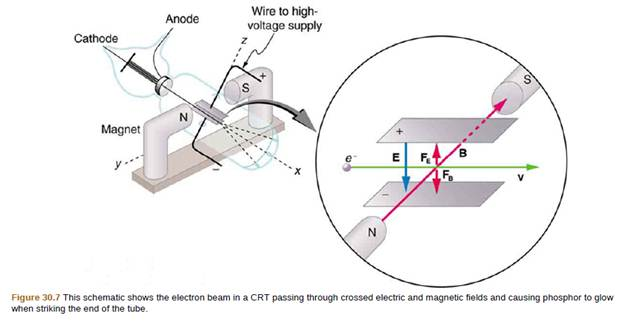Chapter 30, Problem 52PE

Chapter
Section
Textbook Problem

Integrated Concepts The electric and magnetic forces on an electron in the CRT in Figure 30.7 are supposed to be in opposite directions. Verify this by determining the direction of each force for the situation shown. Explain how you obtain the directions (that is, identify the rules used).To determine

Verify the direction of each force for the situation shown and explain how one obtain the directions.

Explanation

Given Data:

The electric and magnetic forces on an electron in the CRT in the below figure are supposed to be in opposite directions.

Formula Used:

The RHR-1 is used to find out the direction of force experienced by a conductor carrying current placed in a magnetic field. The rule states that thumb indicates the direction of the current, while the fingers indicate the direction of magnetic field and the direction which is perpendicular to the palm is the direction of the force.

Calculation:

The figured shows that the electric field is emerging from the positive plate and is terminating at the negative plate. Thus, the direction of electric field is in downward direction.

Also, the magnetic field is emerging from the North pole of one magnet and is travelling towards South pole of other...

Still sussing out bartleby?

Check out a sample textbook solution.

See a sample solution

The Solution to Your Study Problems

Bartleby provides explanations to thousands of textbook problems written by our experts, many with advanced degrees!

Get Started